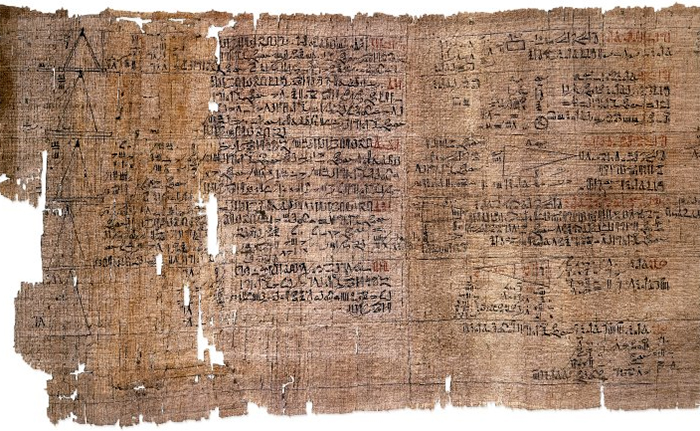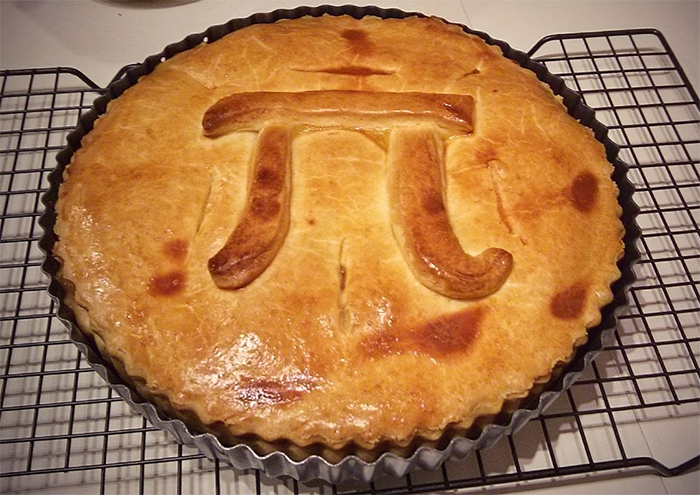(a4ndreas/iStock/Getty Images Plus)

# What Is Pi?

Pi (π) is a constant describing the ratio between a circle's circumference and its diameter. It is an irrational number usually summarized to two decimal places as 3.14.

The Greek letter for P was chosen by the 18th century Welsh mathematics teacher William Jones, most likely to stand for 'periphery'.

Symbolically, the term pi was chosen to stand for something more than a mere number. Prior to its use in the early 18th century, the quantity was represented by terms and fractions, none of which adequately reflected an incomprehensible, infinitely long sequence of non-repeating decimals.

Jones might have suspected that "'the exact proportion between the diameter and the circumference can never be expressed in numbers", but it wasn't until the 1760s that the Swiss polymath Johann Lambert came up with a proof of its irrationality.

## When was pi first calculated?

It seems that for as long as we have been playing with circles, we've been aware that it takes roughly three diameters to make up a circumference, no matter the shape's size.

There's even evidence of this ratio among the mathematics of ancient Babylonians, around 4,000 years ago, who understood the perimeter of a hexagon sitting neatly inside a circle was equal to six times its radius, giving us a value of 3.125.

The Rhind Papyrus (below), produced roughly 1650 BCE in Ancient Egypt, claimed if you were to remove "1/9 of a diameter and construct a square upon the remainder; this has the same area as the circle", which equates to a value of 3.16049.(British Museum Department of Ancient Egypt and Sudan/PD)

Archimedes of Syracuse also had a good stab at the problem. Using polygons much as the Babylonians had, multiplying out the sides, he came up with a more theoretical value of between 3 and 1/7, and 3 and 10/71.

## Why is pi so popular?

As a constant for all circles, pi is an axiom – a fundamental principle – that can be used to help describe a broad range of phenomena and concepts throughout physics and geometry.

This makes it useful in a huge range of applications for analyzing and describing the natural world, from the meandering of rivers to the construct of atoms.

Even where circles don't seem immediately involved, pi can make a strange appearance. For example, the probability that any two integers will have no common positive factors – what's described as being relatively prime – is 6/π^2.

Beyond its practical and mathematical applications, pi has captured the public's interest purely out of a sense of poetry and aesthetic. A staff member and physicist at San Francisco's Exploratorium named Larry Shaw noted at a staff retreat in 1988 how the date March 14 reflected the first three numbers of pi, 3.14.

From there a day of celebrating science and mathematics was born:  Pi Day. More than three decades on and the day is celebrated worldwide, with the sharing of pi trivia, mathematical problems, and the baking of the most famous of circular deserts: the pie.(ScienceAlert)

All Explainers are determined by fact checkers to be correct and relevant at the time of publishing. Text and images may be altered, removed, or added to as an editorial decision to keep information current.